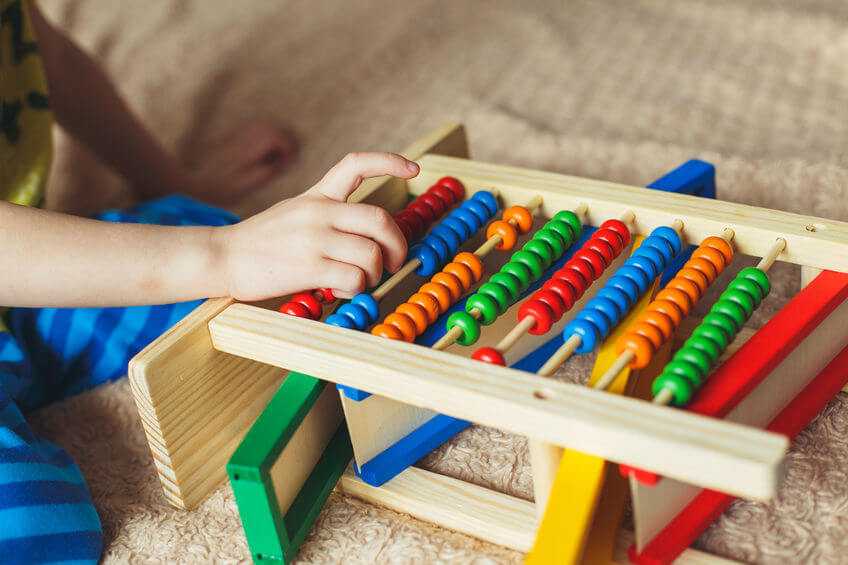# Strategy to teach children to subtract

Subtraction is one of the mathematical operations that children first learn, after addition. It is a very useful operation since it allows them to determine how many elements remains in a set after subtracting a part, without having to recount the whole set. So you can quickly know, for example, how many candies are left after eating a few or how much travel time is missing if 2 hours have passed from the 4 hours planned.In addition, learning to subtract not only will be useful in their daily lives but also help them to solve more complex arithmetic operations later, such as division or equations. A very effective strategy to teach children to subtract is to use educational resources, such as subtraction cards.

Five recommendations to teach children to subtract with subtraction cards:

### 1. Wait for the right moment

To teach children content for those who are not yet prepared can set a negative precedent and make them lose motivation to learn. Therefore, one of the most important aspects to teach them to subtract is to be able to detect the most appropriate moment. In a general sense, children will be prepared to assimilate this content when they already know how to add with relative ease and have matured enough to assimilate new concepts.

### 2. Explain what the subtraction tabs are

Most children like to understand the why of things; in this way they are motivated to discover new concepts. Only if the children understand the usefulness of the subtraction, they will find a meaning in the subtraction cards. Therefore, before showing the children the subtraction cards, it is important that you explain what the subtraction and the subtraction cards consist of, which are nothing more than cards with different subtraction operations.